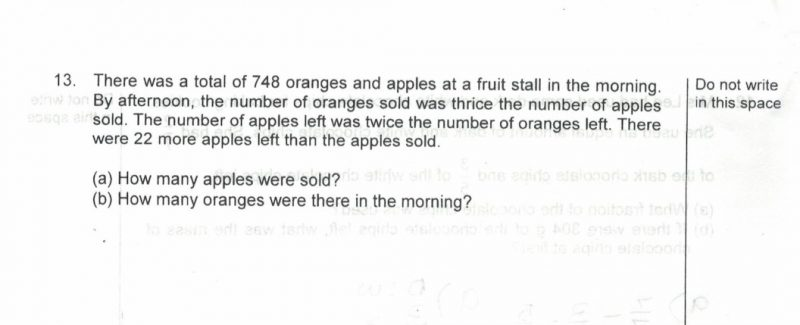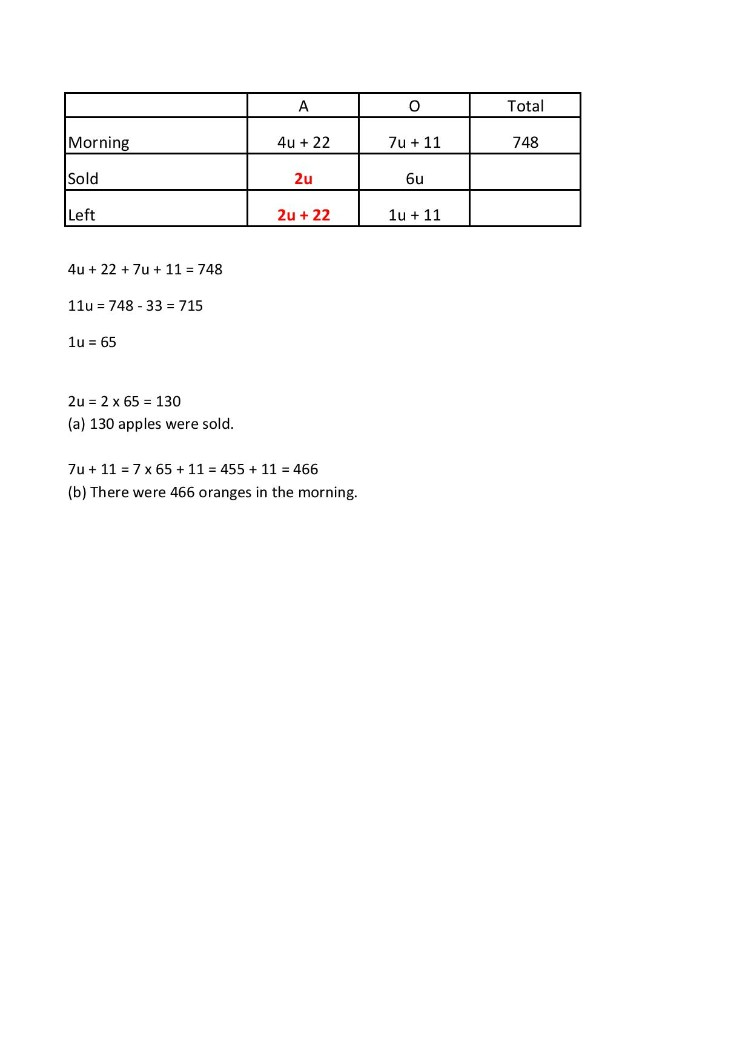# QuestionNeed some help on the above question

Apple sold = 1u

Orange sold = 3u

Apple left = 1u +22 (Number of apples sold + 22)

Apple left is twice the number of orange left

Hence Orange left = 0.5 u +11 {(1u+22)/2}

1u+3u+1u+22+0.5u+11 = 748

5.5u = 748-33

a) 1u = 130 apples

b) 748- (130+130+22) = 466 oranges

Thanks

0 Replies 0 LikesWe first determine the apples left = 2u + 22

Then the oranges left = ½ × (2u + 22) = 1u + 11

Then work backwards to get the apples and oranges in the morning.

0 Replies 1 Like

Hope someone could check my working

Orange.      Apple.    Total

before  7u+11.       4u + 22.       748

sold. (3). 2ux3=6u   (1) 2u

left (1).~1u+11      (2) ~2u+22

i used working  backwards to formulate the at first equation.

a) 7u+11+4u+22=748

11u= 758-33

1u=65

65×2 =130

b) 7x 65+11=466

0 Replies 0 Likes